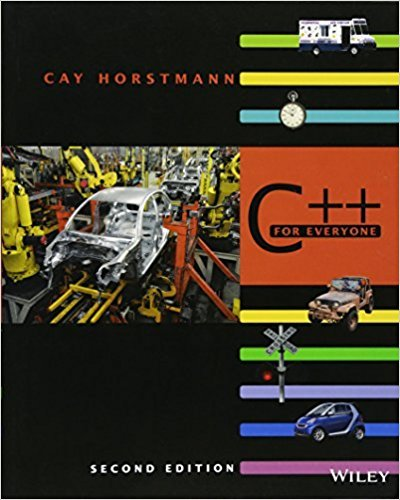×
Get Full Access to C++ For Everyone - 2 Edition - Chapter 5 - Problem P5.16
Get Full Access to C++ For Everyone - 2 Edition - Chapter 5 - Problem P5.16

×

# Enhance the int_name function so that it works correctly for values < 1,000,000,000ISBN: 9780470927137 356

## Solution for problem P5.16 Chapter 5

C++ for Everyone | 2nd Edition

• Textbook Solutions
• 2901 Step-by-step solutions solved by professors and subject experts
• Get 24/7 help from StudySoup virtual teaching assistantsC++ for Everyone | 2nd Edition

4 5 1 358 Reviews
21
4
Problem P5.16

Enhance the int_name function so that it works correctly for values < 1,000,000,000.

Step-by-Step Solution:
Step 1 of 3

Chapter 9 9-1 {4- \r/3=o'oo77Re \kr"prF) ro'o 4#1"'=ooorrlw]" n=ff16.oo71rto'|+r

Step 2 of 3

Step 3 of 3

##### ISBN: 9780470927137

This full solution covers the following key subjects: . This expansive textbook survival guide covers 10 chapters, and 515 solutions. The answer to “Enhance the int_name function so that it works correctly for values < 1,000,000,000.” is broken down into a number of easy to follow steps, and 13 words. The full step-by-step solution to problem: P5.16 from chapter: 5 was answered by , our top Engineering and Tech solution expert on 03/08/18, 08:39PM. Since the solution to P5.16 from 5 chapter was answered, more than 342 students have viewed the full step-by-step answer. This textbook survival guide was created for the textbook: C++ for Everyone , edition: 2. C++ for Everyone was written by and is associated to the ISBN: 9780470927137.

## Discover and learn what students are asking

Calculus: Early Transcendental Functions : The Natural Logarithmic Function: Integration
?In Exercises 1-26, find the indefinite integral. $$\int \frac{1}{x+1} d x$$

Chemistry: The Central Science : Gases
?Complete the following table for an ideal gas:

Statistics: Informed Decisions Using Data : Two-Way Analysis of Variance
?To graphically display the role interaction plays in a factorial design, we draw ______.

Unlock Textbook Solution